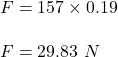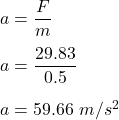Question

A 0.50 kg object is attached to a spring with force constant 157 N/m so that the object is allowed to move on a horizontal frictionless surface. The object is released from rest when the spring is compressed 0.19 m.(a) Find the force on the object.1 N
(b) Find its acceleration at that instant 2 m/s2

1.Thunguyet

Explanation:

We have,

Mass of an object is 0.5 kg

Force constant of the spring is 157 N/m

The object is released from rest when the spring is compressed 0.19 m.

(A) The force acting on the object is given by :

F = kx(B) The force is simply given by :

F = ma

a is acceleration at that instant